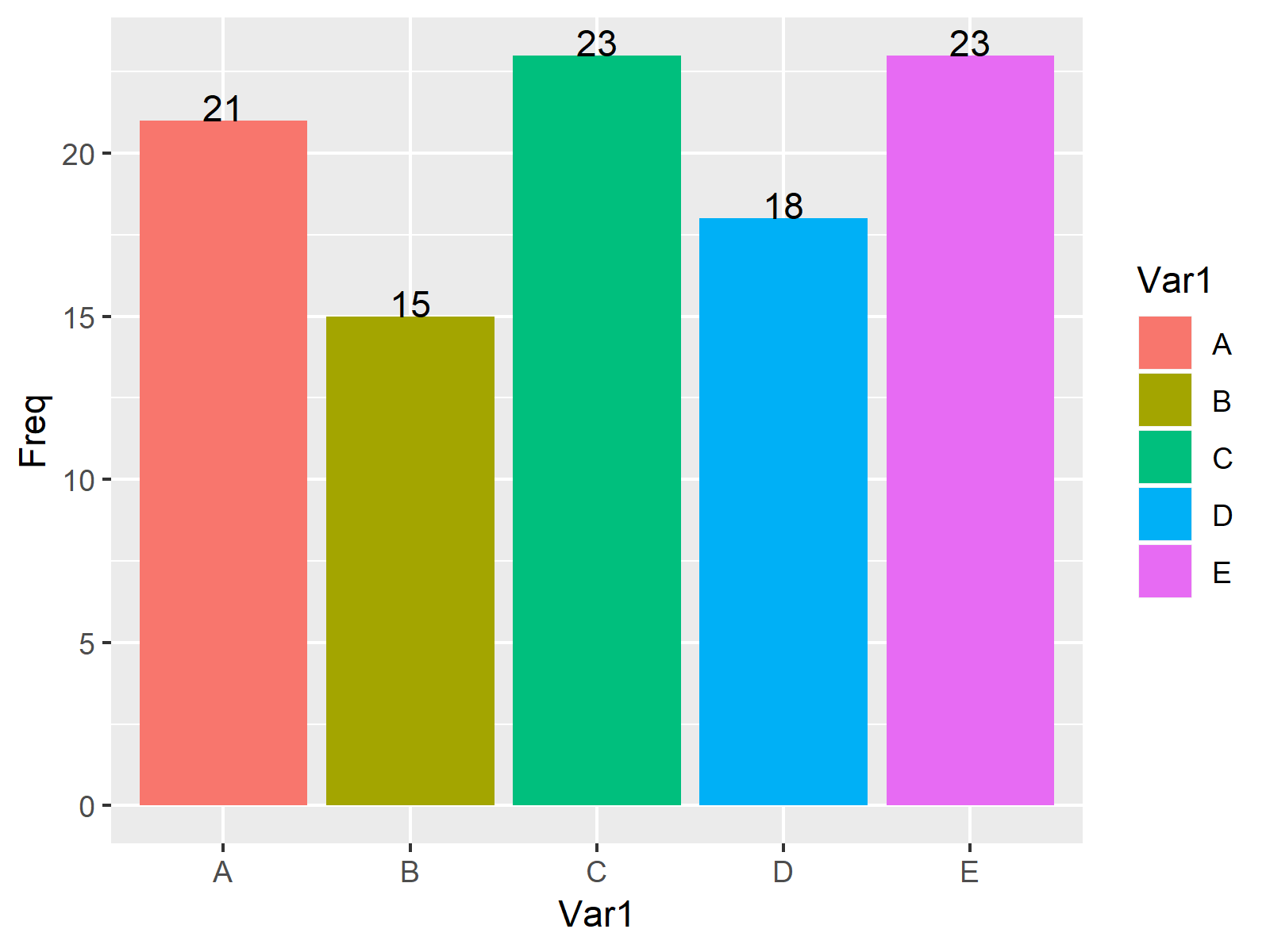# Add Count Labels on Top of ggplot2 Barchart in R (Example)

In this tutorial you’ll learn how to add the frequency count on the top of each bar of a ggplot2 barchart in R.

## Example Data, Packages & Basic Plot

We’ll use the following data as basement for this R tutorial:

```set.seed(983274)                                          # Create random example data
data <- data.frame(x = sample(LETTERS[1:5], 100, replace = TRUE))
head(data)                                                # Print first lines of data
# x
# 1 D
# 2 C
# 3 B
# 4 B
# 5 C
# 6 C```

Have a look at the previously shown output of the RStudio console. It shows that our example data has only one column containing alphabetic letters.

For the following R syntax, we also have to install and load the ggplot2 package:

```install.packages("ggplot2")                                 # Install & load ggplot2 package
library("ggplot2")```

Finally, let’s move on to the example code…

## Example: Drawing Barplot with Values on Top

In this Example, I’ll explain how to plot frequency labels on top of each bar of a ggplot2 barplot. First, we need to compute the frequency count within each group of our data:

```data_srz <- as.data.frame(table(data\$x))                  # Summarize data
data_srz                                                  # Print summarized data
#   Var1 Freq
# 1    A   21
# 2    B   15
# 3    C   23
# 4    D   18
# 5    E   23```

In the output of the RStudio console above, you can see how often each group is contained in our example data. Now, we can plot our data in a barchart with counting labels on top as shown below:

```ggplot(data_srz, aes(x = Var1, y = Freq, fill = Var1)) +  # Plot with values on top
geom_bar(stat = "identity") +
geom_text(aes(label = Freq), vjust = 0)```As visualized in Figure 1, the previously shown R programming syntax created a barplot with counts on top of each bar with the ggplot2 package.

## Video, Further Resources & Summary

Do you want to learn more about drawing labels on top of bars? Then I can recommend watching the following video of my YouTube channel. In the video, I illustrate the R codes of this tutorial.

Please accept YouTube cookies to play this video. By accepting you will be accessing content from YouTube, a service provided by an external third party.If you accept this notice, your choice will be saved and the page will refresh.

Furthermore, I can recommend having a look at some of the other tutorials of this website. I have published several tutorials already:

To summarize: At this point you should know how to print the count labels of each bar on the top of the barchart in the R programming language. Let me know in the comments section below, in case you have any additional questions.

Subscribe to the Statistics Globe Newsletter

•rick
September 9, 2022 9:10 pm

How would you handle this if you had multiple entries for A, B, C etc? I am getting stacked numbers on the bars because there are multiple rows with data (the rows denote users, the X is a factor, and the Y is a total per user).

•September 19, 2022 11:06 am